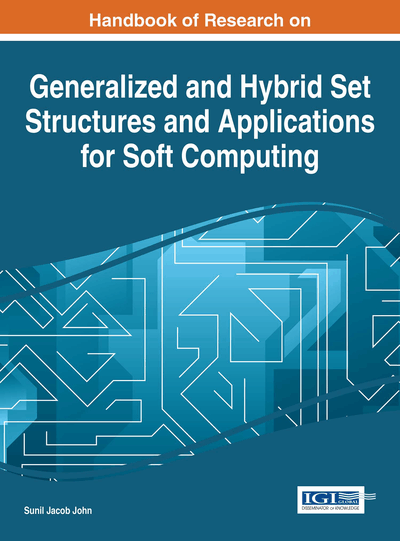# Introduction to Intuitionistic Fuzzy Multisets and Its Applications

T. K. Shinoj (National Institute of Technology Calicut, India) and Sunil Jacob John (National Institute of Technology Calicut, India)
DOI: 10.4018/978-1-4666-9798-0.ch003

## Abstract

In this chapter a new concept named Intuitionistic Fuzzy Multiset is introduced, which is an attempt to combine the two concepts: Intuitionistic Fuzzy sets and Fuzzy Multisets. The basic operations and their various properties are discussed. The authors discussed two significant applications of Intuitionistic Fuzzy Multisets. Most of human reasoning involves the use of variables whose values are fuzzy sets. This is the basis for the concept of a linguistic variable. But in some situations like decision making problems, the description by a linguistic variable in terms of membership function only is not adequate. There is chance of existing a non-null complement. There are situations that each element has different membership values. In such situations Intuitionistic Fuzzy Multisets is more adequate. Here the authors present Intuitionistic Fuzzy Multisets as a tool for reasoning such a situation through a medical diagnosis problem. As the second application, accuracy of Collaborative Robots using the concept of Intuitionistic Fuzzy Multiset is discussed.
Chapter Preview
Top

## 1. Introduction

Modern set theory formulated by George Cantor is fundamental for the whole Mathematics. One issue associated with the notion of a set is the concept of vagueness. Mathematics requires that all mathematical notions including set must be exact. This vagueness or the representation of imperfect knowledge has been a problem for a long time for philosophers, logicians and mathematicians. However, recently it became a crucial issue for computer scientists, particularly in the area of artificial intelligence. To handle situations like this, many tools were suggested. They include Fuzzy sets, Multi sets, Rough sets, Soft sets and many more.

Considering the uncertainty factor, Lotfi Zadeh (1965) introduced Fuzzy sets, in which a membership function assigns to each element of the universe of discourse, a number from the unit interval [0,1] to indicate the degree of belongingness to the set under consideration. Krassimir. T. Atanassov (1983,1986) introduced the concept of Intuitionistic Fuzzy sets (IFS) by introducing a non-membership function together with the membership function of the fuzzy set. Among the various notions of higher-order Fuzzy sets, Intuitionistic Fuzzy sets proposed by Atanassov provide a flexible framework to explain uncertainty and vagueness. IFS reflect better the aspects of human behavior.

A human being who expresses the degree of belongingness of a given element to a set, does not often expresses the corresponding degree of non-belongingness as the complement. This psychological fact states that linguistic negation does not always coincides with logical negation. This idea of Intuitionistic fuzzy sets, which is a natural generalization of a standard Fuzzy set, seems to be useful in modeling many real life situations, like negotiation processes, psychological investigations, reasoning etc. The relation between Intuitionistic Fuzzy sets and other theories modeling imprecision are discussed by Glad Deschrijver, Etienne (2007) and Takeuti, Titani (1984).

Many fields of modern mathematics have been emerged by violating a basic principle of a given theory only because useful structures could be defined this way. Set is a well-defined collection of distinct objects, that is, the elements of a set are pair wise different. If we relax this restriction and allow repeated occurrences of any element, then we can get a mathematical structure that is known as Multisets or Bags. For example, the prime factorization of an integer n>0 is a Multiset whose elements are primes. The number 120 has the prime factorization 120 = 233151 which gives the Multiset {2, 2, 2, 3, 5}. A complete account of the development of multiset theory are discussed by Blizard (1989,1993). As a generalization of multiset, Yager (1986) introduced the concept of Fuzzy Multiset (FMS). An element of a Fuzzy Multiset can occur more than once with possibly the same or different membership values. A complete account of the applications of Fuzzy Multisets in various fields can be seen in Cristian S. Calude, Gheorghe Paun, Grzegorz Rozenberg and Arto Salomaa (2001).

This chapter is an attempt to combine the two concepts: Intuitionistic Fuzzy sets and Fuzzy Multisets together by introducing a new concept called Intuitionistic Fuzzy Multisets (IFMS) by Shinoj T K, Sunil Jacob John (2012). In the same papers the authors discussed an application in Medical diagnosis. An application in Robotics is discussed by Shinoj T K, Sunil Jacob John (2013), in which accuracy of Collaborative Robots using the concept of Intuitionistic Fuzzy Multiset is explained. Some of the basic operation on Intuitionistic Fuzzy Multiset is given in the next section. More detailed basic operations are available in Shinoj T K & Sunil Jacob John (2013 November).

## Complete Chapter List

Search this Book:
Reset